# Test: Angle Subtended By The Chord

## 20 Questions MCQ Test Mathematics (Maths) Class 9 | Test: Angle Subtended By The Chord

Description
This mock test of Test: Angle Subtended By The Chord for Class 9 helps you for every Class 9 entrance exam. This contains 20 Multiple Choice Questions for Class 9 Test: Angle Subtended By The Chord (mcq) to study with solutions a complete question bank. The solved questions answers in this Test: Angle Subtended By The Chord quiz give you a good mix of easy questions and tough questions. Class 9 students definitely take this Test: Angle Subtended By The Chord exercise for a better result in the exam. You can find other Test: Angle Subtended By The Chord extra questions, long questions & short questions for Class 9 on EduRev as well by searching above.
QUESTION: 1

Solution:
QUESTION: 2

Solution:
QUESTION: 3

### If the angles subtended by the chords of a circle at the centre are equal, then the chords are

Solution:
QUESTION: 4

Name the chord that subtends angle FOE at the centre.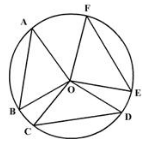Solution:
QUESTION: 5

In the given figure name the angle subtended by the chord PQ on the major arc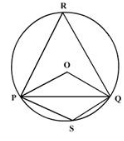Solution:
QUESTION: 6

In figure, O and O” are centres of two circles intersecting at B and C. ACD is a straight line, then the value of x is: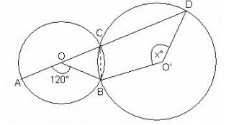Solution:
QUESTION: 7

The angles subtended by arcs XY and MN on the centre of the circle are 72° each. The length of chord XY = 15 cm, then the length of chord MN is:

Solution:
QUESTION: 8

In a circle with center O and a chord BC, points D and E lie on the same side of BC. Then, if∠BDC=80°, then ∠BEC =

Solution:
QUESTION: 9

In a circle with center O and a chord BC, the point D lies on the same side BC as O. If ∠ BOC = 50°,then ∠ BDC =

Solution:
QUESTION: 10

A regular octagon is inscribed in a circle. The angle that each side of the octagon subtends at the centre is

Solution:
QUESTION: 11

In the given figure, what is the measurement of YXZ?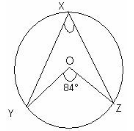Solution:
QUESTION: 12

In figure, O is the centre of the circle and PS bisects ∠QPR. Find ∠QRS.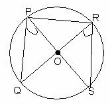Solution:
QUESTION: 13

An equilateral triangle ABC is inscribed in a circle with centre O. Angle BOC will be

Solution:
QUESTION: 14

In figure arc PQ = arc PR, ∠POQ = 30° and ∠QOS = 70°. Find ∠ROS.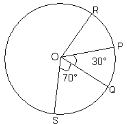Solution: Sol^ :- Join RP and PQ. then, RP=PQ [ Since *arc RP=arcPQ* ] Now since segment RP=PQ Then, ∠POR =∠POQ = 30* [ Angles of same or equal segment ] Now, ∠ROS = ∠POQ+∠POR+∠QOS ∠ROS = 30*+30*+70* ∠ROS = 130* 《* represents DEGREE》
QUESTION: 15

Chord AB subtends ∠AOB = 60° at centre. If OA = 5 cm then length of AB (in cm) is :

Solution:
QUESTION: 16

In the figure, ∠M = 82°, then ∠O = ?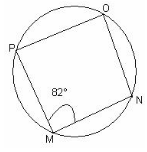Solution:
QUESTION: 17

If ∠OAB = 40° then ∠ACB is equal to: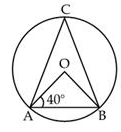Solution:

In ΔQAB, OA = OB [both are the radius of a circle]
∠OAB = ∠OBA ⇒ ∠OBA = 40°
[angles opposite to equal sides are equal] Also, ∠AOB + ∠OBA + ∠BAO = 180°
[by angle sum property of a triangle]
∠AOB + 40° + 40° = 180°
⇒ ∠AOB = 180° – 80° = 100°
We know that, in a circle, the angle subtended by an arc at the centre is twice the angle subtended by it at the remaining part of the circle.
∠AOB = 2 ∠ACB ⇒ 100° =2 ∠ACB
∠ACB = 100°/2 = 50°

QUESTION: 18

In the figure, if AB is the diameter of the circle, then the value of x is: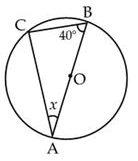Solution:
QUESTION: 19

An equilateral ΔABC is inscribed in a circle with centre O. The measure of ∠BOC is:

Solution:
QUESTION: 20

If the regular polygon is inscribed in a circle the sum of all the angles are at the the centre is

Solution: